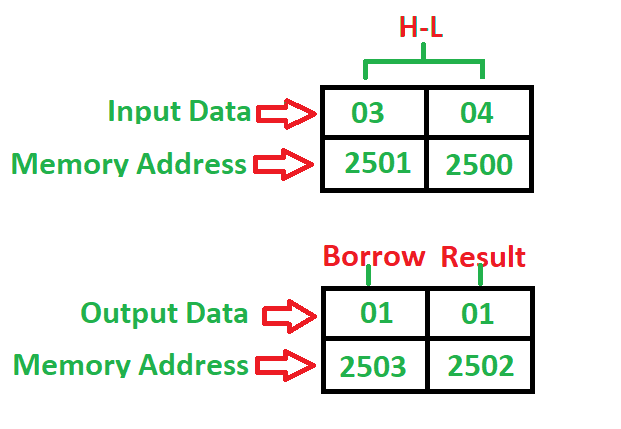# 8085 program to subtract two 8-bit numbers with or without borrow

Problem – Write a program to subtract two 8-bit numbers with or without borrow where first number is at 2500 memory address and second number is at 2501 memory address and store the result into 2502 and borrow into 2503 memory address. Example –Algorithm –

1. Load 00 in a register C (for borrow)
2. Load two 8-bit number from memory into registers
3. Move one number to accumulator
4. Subtract the second number with accumulator
5. If borrow is not equal to 1, go to step 7
6. Increment register for borrow by 1
7. Store accumulator content in memory
8. Move content of register into accumulator
9. Store content of accumulator in other memory location
10. Stop

Program –

Explanation – Registers A, H, L, C are used for general purpose:

1. MOV is used to transfer the data from memory to accumulator (1 Byte)
2. LHLD is used to load register pair directly using 16-bit address (3 Byte instruction)
3. MVI is used to move data immediately into any of registers (2 Byte)
4. STA is used to store the content of accumulator into memory(3 Byte instruction)
5. INR is used to increase register by 1 (1 Byte instruction)
6. JNC is used to jump if no borrow (3 Byte instruction)
7. SUB is used to subtract two numbers where one number is in accumulator(1 Byte)
8. HLT is used to halt the program

• The program is a simple and efficient way to subtract two 8-bit numbers with or without borrow using the 8085 microprocessor.

• The program uses only a few instructions and requires minimal memory space, making it easy to implement in a microcontroller.

• The program produces accurate results since it handles both with and without borrow conditions using conditional jump and complement instructions.

• The program can be easily modified to subtract larger or smaller numbers by changing the memory addresses.